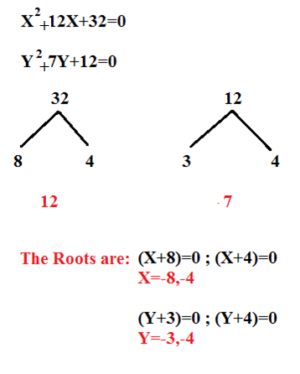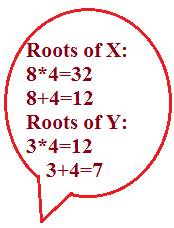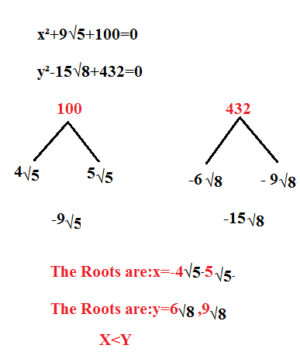# LET US SEE HOW TO SOLVE QUADRATIC EQUATION-HOW TO SERIES

Hi Bankersdaily Aspirants,

Aspirants today we are going to discuss on the most important topic in Aptitude Section that is Quadratic Equation.Quadratic Equation is asked for 5 marks in Prelims & Mains Examination.This is the easiest section where we can score 5 marks in less than 5 minutes.In this Article we are going to discuss some of the pattern following which Question on Quadratic Equation  is asked.

TOPICS TO BE DISCUSSED

2)BASED ON ROOTS

3)BASED ON FINDING VALUE

## #.1.TYPE 1:

### BASED ON BASIC QUADRATIC EQUATION:

Find the relationship between X & Y.

1)x²-12x+32=0

y²-7x+12=0

EXPLANATIONX≤Y

### PRACTICE THIS SUM :

1)X²+5X+6=0

Y²+12Y+35=0

The Roots of X=-2,-3

The Roots of Y=-5,-7

X>Y

2)2X²-10X+12=0

3Y²-12+9=0

The Roots of X=2,3;

The Roots of Y=1,3

Relationship cannot be established

## #.2. TYPE 2:

### BASED ON ROOTS:

This type of Pattern is asked last year in IBPS PO Mains Examination.

1)x²+9√5+100=0

y²-15√8+432=0### PRACTICE THIS SUM:

1)X²-11√8+240=0

Y²-14√8+360=0

The Roots of X=6√8,5√8

The Roots of Y=5√8,9√8

Cannot be Established

2)X²+11√6+90=0

Y²+15√6+216=0

The Roots of X=-5√6,-6√6

The Roots of Y=-6√6,-9√6

X≥Y

## REMEMBER:

### If you Remember this Table then you can answer without Solving,

 SIGN IN THE GIVEN EQUATION SIGN OF LARGER NUMBER SIGN OF SMALLER NUMBER +,+ – – -,+ + + +,- – + -,- + –

## #.3 TYPE 3:

### BASED ON FINDING VALUE:

In this type you will not be given direct value’s you have to find the value from the given statement and find the relationship between them.Let see with an example,

X=Area of Square whose Side is 4cm

Y=Area of Equilateral Triangle whose side is 8cm

EXPLANATION

X=Area of Square=4²=16 Sq.cm

Y=Area of Equilateral Triangle √3/4 * 8² = 16√3 Sq.cm

Therefore X<Y.

### PRACTICE THIS SUM:

1)X=Area of Triangle whose base is 1.5cm and height is 75cm

Y=Area of the equilateral triangle whose side is 12 cm

Area of Triangle=1/2*b*h=5625 sq.cm

Area of Equilateral Triangle=√3/4*Side²=36√3 Sq.cm

X>Y

2)X=length of the diagonal of a square whose area is 400 sq.m

Y=Perimeter of a Square Plot whose area is 400 sq.m

X=√(2* area)

=20√2metre

Y=√(16*400)

=4*20=80metre

X<Y